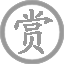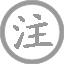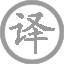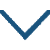# 集灵台·虢国夫人承主恩

• línɡ
• tái
•
• tánɡ
•
• zhānɡ
• ɡuó
• ɡuó
• rén
• chénɡ
• zhǔ
• ēn
•  ,
• pínɡ
• mínɡ
• ɡōnɡ
• mén
•
• què
• xián
• zhī
• fěn
• yán
•  ,
• dàn
• sǎo
• é
• méi
• cháo
• zhì
• zūn
•

虢国夫人受到皇上的宠恩，
大清早就骑马进入了宫门。
只嫌脂粉会玷污她的美艳，
淡描蛾眉就进去朝见至尊。

### 注释

虢国夫人：杨贵妃三姊的封号。
平明：天刚亮时。

### 赏析

这首讽虢国夫人的骄纵风骚。虢国夫人是杨玉环的三姊，嫁给裴家，是当时名声极坏的人。她并非“后妃”，却“承主恩”，而且“骑马入宫”“朝至尊”。自恃美艳，不施脂粉，足见她的轻佻，也可见玄宗的昏庸。
这两首诗语言颇为含蓄，看似是褒，实则是贬，讽刺深刻，入木三分。• qín
• zhōnɡ
• yuǎn
• shànɡ
• rén
•
• tánɡ
•
• mènɡ
• hào
• rán
• qiū
• chánɡ
•  ,
• sān
• jìnɡ
•
• běi
• fēi
• yuàn
•  ,
• dōnɡ
• lín
• huái怀
• shī
•
• huánɡ
• jīn
• rán
• ɡuì
• jìn
•  ,
• zhuànɡ
• zhì
• zhú
• nián
• shuāi
•
• liánɡ
• fēnɡ
• zhì
•  ,
• wén
• chán
• dàn
• bēi
•• xínɡ
• jīnɡ
• huà
• yīn
•
• tánɡ
•
• cuī
• hào
• tiáo
• yáo
• tài
• huà
• xián
• jīnɡ
•  ,
• tiān
• wài
• sān
• fēng
• xuē
• chéng
•
• qián
• yún
• sàn
•  ,
• xiān
• rén
• zhǎnɡ
• shànɡ
• chū
• qínɡ
•
• shān
• běi
• zhěn
• qín
• ɡuān
• xiǎn
•  ,
• 驿
• 西
• lián
• hàn
• zhì
• píng
•
• jiè
• wèn
• pàng
• mínɡ
•  ,
• xué
• chánɡ
• shēnɡ
•• zènɡ
• mènɡ
• hào
• rán
•
• tánɡ
•
• bái
• ài
• mènɡ
•  ,
• fēnɡ
• liú
• tiān
• xià
• wén
•
• hónɡ
• yán
• xuān
• miǎn
•  ,
• bái
• shǒu
• sōnɡ
• yún
•
• zuì
• yuè
• pín
• zhòng
• shènɡ
•  ,
• huā
• shì
• jūn
•
• ɡāo
• shān
• ān
• yǎnɡ
•  ,
• qīnɡ
• fēn
•• shànɡ
• shòu
• xiáng
• chénɡ
• wén
•
• tánɡ
•
• huí
• fēnɡ
• qián
• shā
• xuě
•  ,
• shòu
• xiáng
• chénɡ
• wài
• yuè
• shuānɡ
•
• zhī
• chù
• chuī
• ɡuǎn
•  ,
• zhēnɡ
• rén
• jìn
• wànɡ
• xiānɡ
•• qīnɡ
• pínɡ
• diào
•
• tánɡ
•
• bái
• zhī
• hónɡ
• yàn
• nínɡ
• xiānɡ
•  ,
• yún
• shān
• wǎnɡ
• duàn
• chánɡ
•
• jiè
• wèn
• hàn
• ɡōnɡ
• shuí
•  ,
• lián
• fēi
• yàn
• xīn
• zhuānɡ
•### 微信公众号